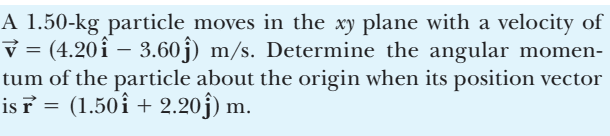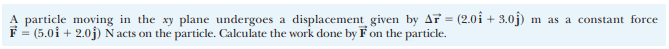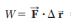# Difference between scalar and cross product

Callumnc1
Homework Statement:
I am interested to understand how to tell when to use the scalar or cross product when solving physics problems.
Relevant Equations:
Instantaneous angular momentum = cross product of the instantaneous position vector and instantaneous momentum
Hi!

For example, how do you tell whether to use the scalar or cross product for an problem such as,However, I do know that instantaneous angular momentum = cross product of the instantaneous position vector and instantaneous momentum. However, what about if I didn't know whether I'm meant to take the cross product or scalar product of the quanties given. For example, for this work problem,How would I tell whether to use the scalar or dot product just by looking at the work equation,Many thanks!

Last edited:

Homework Helper
Gold Member
2022 Award
How would I tell whether to use the scalar or dot product just by looking at the work equation,
View attachment 317934
The equation you posted shows ##\cdot##, the dot (or 'scalar') product operator. If it were the cross (or 'vector') product it would show ##\times## as the operator.

•Callumnc1 and Orodruin
Staff Emeritus
Homework Helper
Gold Member
How would I tell whether to use the scalar or dot product just by looking at the work equation,The work equation is defined using the dot product, just as angular momentum is defined with a cross product.

•Callumnc1
Callumnc1

Homework Helper
Gold Member
Given two 3D vectors, ##\mathbf{A}## and ##\mathbf{B}##, in component form, and you were asked to find the angle between them, you could use either the dot product or the cross product. First you find the magnitudes, ##A## and ##B##, using the Pythagorean theorem in 3D. Then,
$$\cos\theta=\frac{\mathbf{A}\cdot\mathbf{B}}{AB}\implies \theta=\arccos\left[\frac{\mathbf{A}\cdot\mathbf{B}}{AB}\right].$$ Note that if the dot product is negative, the angle is greater than 90°, because ##\cos(\pi-\theta)=-\cos\theta.##

You could also use, $$\sin\theta=\frac{\|\mathbf{A}\times\mathbf{B}\|}{AB}\implies \theta=\arcsin\left[\frac{\|\mathbf{A}\times\mathbf{B}\|}{AB}\right].$$ However, I do not recommend this method for two reasons: (a) an additional calculation is needed to find the magnitude of the cross product vector ##\|\mathbf{A}\times\mathbf{B}\|##; (b) one has to draw the vectors tail-to-tail to figure out whether the angle is less or greater than 90° because ##\sin(\pi-\theta)=+\sin\theta##. More work means increased likelihood for mistakes.

•Callumnc1
Callumnc1
Given two 3D vectors, ##\mathbf{A}## and ##\mathbf{B}##, in component form, and you were asked to find the angle between them, you could use either the dot product or the cross product. First you find the magnitudes, ##A## and ##B##, using the Pythagorean theorem in 3D. Then,
$$\cos\theta=\frac{\mathbf{A}\cdot\mathbf{B}}{AB}\implies \theta=\arccos\left[\frac{\mathbf{A}\cdot\mathbf{B}}{AB}\right].$$ Note that if the dot product is negative, the angle is greater than 90°, because ##\cos(\pi-\theta)=-\cos\theta.##

You could also use, $$\sin\theta=\frac{\|\mathbf{A}\times\mathbf{B}\|}{AB}\implies \theta=\arcsin\left[\frac{\|\mathbf{A}\times\mathbf{B}\|}{AB}\right].$$ However, I do not recommend this method for two reasons: (a) an additional calculation is needed to find the magnitude of the cross product vector ##\|\mathbf{A}\times\mathbf{B}\|##; (b) one has to draw the vectors tail-to-tail to figure out whether the angle is less or greater than 90° because ##\sin(\pi-\theta)=+\sin\theta##. More work means increased likelihood for mistakes.
Thank you kuruman for your answer! It is Interesting to know how to find the angle between vectors using the cross and scalar product.

•kuruman